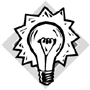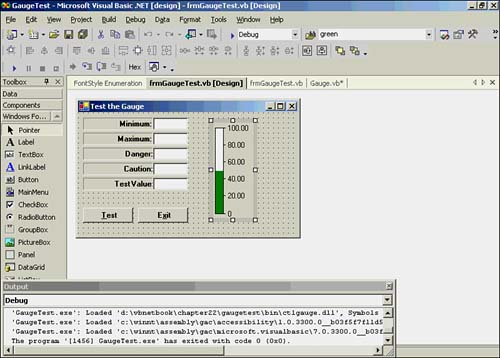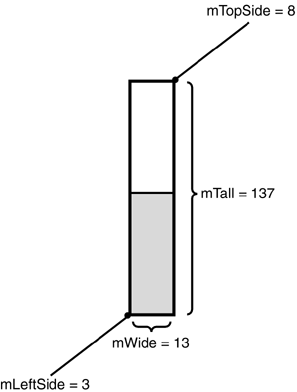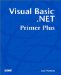# Using the Yellowline and Redline Features

### Using the Yellowline and Redline Features

The If statement block is used to determine the fill color for the gauge:

` If mCaution <> 0 Or mDanger <> 0 Then ' have they set yellow or red lines?   If mCurrent >= mCaution Then    ' See if value requires a color change   MyBrush.Color = Color.Yellow  End If  If mCurrent >= mDanger Then   MyBrush.Color = Color.Red  End If End If `

If either mCaution or mDanger is nonzero, you can assume that the user wants to activate the yellowline or redline features of the gauge. If the current value ( mCurrent ) is greater than mCaution , the brush color is set to yellow. If the current value is greater than mDanger , the color is set to red.

Programmer's TipAlthough the If statement block is not RDC (really dumb code), it does qualify as DC (doofus code) quality. This is because if mCurrent is greater than or equal to mDanger , you set the brush color twice. The old adage "If it ain't broke, don't fix it" is sage advice in most cases. However, you can remove the possibility of double-setting the brush color by making a trivial change in the code. You should make this change to the code before you show it to your friends at the next cocktail party. (I've written a lot of code thus far it's time for you to chip in.)

Figure 22.9 shows a screen shot of the gauge that should give you a better idea of how the rectangles look.

##### Figure 22.9. The Gauge control at design time, using the defaults.To better understand how the gauge is actually drawn, the most important elements of the gauge are labeled in Figure 22.10. The control size is 62x152 pixels for the gauge shown in Figure 22.9. If you look at the code for the Gauge_Resize() event in Listing 22.5 and use the control size of 62x152, you find the following:

` mTall   = 152 * .9    = 137  mTopSide = (152  137) / 2 = 8 mLeftSide = 62 * .05    = 3 mWide   = 62 * .2     = 13 `
##### Figure 22.10. Important measurements on the Gauge control.Don't forget that these are pixel ( Integer ) values, so Visual Basic .NET rounds each to the next whole pixel.

#### Drawing and Filling the Background of a Gauge

When the brush color is set based on the value of mCurrent relative to the yellowline and redline colors, you call DrawRectangle() to draw the outline of the gauge. This statement:

` Canvas.DrawRectangle(MyPen, mLeftSide, mTopSide, mWide, mTall) `

translates to this:

` Canvas.DrawRectangle(MyPen, 3, 8, 13, 137) `

using the numbers from Figure 22.10. As you can see from the DrawRectangle() statement, the first argument is the pen used to draw the rectangle. The next two arguments are the x, y-coordinates for the upper-left corner of the gauge (3,8). The next argument ( 13 ) is the width of the rectangle you are drawing, and the final argument ( 137 ) is its height. When you finish processing the DrawRectangle() statement, the outline of the gauge is drawn on the Gauge control.

The next statement is used to fill the rectangle:

` Canvas.FillRectangle(MyBrush, mLeftSide + 1, mTopSide + 1, mWide - 1, mTall - 1) `

Again, the FillRectangle() method uses the same coordinate system as DrawRectangle() , with a few minor exceptions. If you have done some graphics programming before, you know that there is a process called flood fill. A flood fill method passes in an x,y-coordinates location, a border color (for example, black), and a fill color (for example, green). The method then proceeds to check the pixels around its current location. If the color is the border color, the pixel is not changed. If the color is not the border color, the color is changed to the fill color. The method then changes the coordinates and checks its neighboring pixel colors and repeats the process. When the method is finished, the interior of the object should have been filled in with the fill color.

The problem is that a flood fill spends a lot of time relocating itself and checking pixel values. The advantage of a FillRectangle() method is that it doesn't have to perform the border checking tests; it just blasts the color by using the parameters passed to it.

In the call to FillRectangle() , you offset each parameter by 1 pixel. The first two parameters (the x,y-coordinates) are each increased by 1 pixel. This has the effect of locating the starting position for the new rectangle just inside the border of the existing gauge. You subtract 1 pixel from the width and height of the gauge. If you think about it, this results in a new rectangle that fits inside the gauge you just drew with the DrawRectangle() call. Therefore, this process preserves the black border of the Gauge control.

#### Drawing the Tick Marks and Values

The next four statements calculate some values that are needed to draw the tick marks on the gauge and label them:

` MyBrush.Color = Color.Black      ' Reset brush color for numbers  TickOffset = mWide + mLeftSide TickValues = mMax NumberOffset = MyFont.GetHeight(Canvas) / 2 `

The first statement resets the MyBrush color from the background color of the gauge (that is, green, yellow, or red) to black.

If you look at Figure 22.10 and fill in the appropriate values, you should find that TickOffset results in an x-coordinate value that is just on the right edge of the gauge. TickValues is initialized to mMax . In terms of Figure 22.9, TickValues would be set to 100 .

NumberOffset is used to center the numeric values on the tick marks. The GetHeight() method call returns the height (in pixels) for the font you are using. On my system, the value is 12 pixels, so any character that is part of the Microsoft Sans Serif font will fit within a vertical distance of 12 pixels for the font size used. You divide the return value in half and assign it into NumberOffset . By subtracting NumberOffset from the y-coordinate for each character you draw, you locate each string value one-half character higher on the gauge. Using this offset has the effect of centering the numbers on their respective tick marks. You can see the effect in Figure 22.9.

Consider what this For loop from Listing 22.6 does:

` For i = 0 To 4            ' Draw the tick marks and values   TopOffset = mTopSide + i * mGap   ' First, draw tick marks  Canvas.DrawLine(MyPen, TickOffset, TopOffset, TickOffset + 3, TopOffset)  buff = Format(TickValues - mTick * i, ".00")  ' Now draw scale values  Canvas.DrawString(buff, MyFont, MyBrush, TickOffset + 5, _             TopOffset - NumberOffset) Next i `

The first statement in this For loop calculates where a tick mark should be drawn. For the dimensions you've been using, you should see that mGap equals 27 pixels. The call to the Drawline() method when i is set to looks like this:

` 8 = 8 + 0 * 27 Canvas.DrawLine(MyPen, 16, 8, 11, 8) `

The first tick mark (which is a 3-pixel line) is drawn just in front of the value 100.00 in Figure 22.9. On the second pass through the For loop, i should equal 1 , so the values change to this:

` 35 = 8 + 1 * 27 Canvas.DrawLine(MyPen, 16, 35, 11, 35) `

This line becomes the tick mark for the value 80.00 in Figure 22.9. You can check the other values as additional passes are made through the loop.

Now you should look that the calculation of buff :

` buff = Format(TickValues - mTick * i, ".00")  ' Now draw scale values `

Recall that TickValues was initialized to mMax , or 100 . mTick is the range of values (range = mMax mMin = 100) divided by 5. Therefore, mTick is 20 . On the first pass through the loop, buff becomes this:

` "100.00" = Format(100 - 20 * 0, ".00") `

On the second pass, the calculation becomes this:

` "80.00" = Format(100 - 20 * 1, ".00") `

I think you get the idea.

The DrawString() method call:

` Canvas.DrawString(buff, MyFont, MyBrush, TickOffset + 5, TopOffset - NumberOffset) `

draws the contents of buff 5 pixels to the right of the edge of the control (that is, the x- coordinate equals TickOffset + 5). The y-coordinate is set by using the calculated value of TopOffset minus the font offset (that is, NumberOffset ).

When the For loop finishes, all the numeric string values and their associated tick marks appear on the control. The final calls to DrawLine() and DrawString() are done outside the loop because you want to align the last tick mark with the bottom of the gauge. Because of rounding errors in calculating the offsets in the loop, the final tick mark might not line up perfectly if you drew it by using the For loop calculations. (The technical term for this kind of code is fudging code. Not elegant, but necessary.)

Finally, you set the MyBrush color to white in preparation for drawing the gauge background color. The calculation of i in this statement is used to determine the y-coordinate value for the new rectangle:

` i = (1.0 - mCurrent / mRange) * mTall + (mMin / mRange) * mTall  1 `

For example, if the current value, mCurrent , is 75 and using the gauge shown in Figure 22.9, the numbers look like this:

` i = (1.0 - 75 / 100) * 137 + (0 / 100) * 137  1  i = (.25) * 137 + (0) * 137  1 i = 34.25 + 0  1 i = 33.25 `

which means i equals 33 because it is an Integer data type. This value then becomes the y-coordinate value in this statement:

` Canvas.FillRectangle(MyBrush, mLeftSide + 1, mTopSide + 1, mWide - 1, i) `

The call to FillRectangle() fills in the gauge with the white color held in MyBrush . Because the entire Gauge control was previously filled in with appropriate gauge value color (that is, green, yellow, or red, depending on mCurrent ), the new white rectangle is actually drawing the background color for the gauge based on mCurrent . This gives the appearance of setting the gauge color, when in fact you are setting the background color. If you think about this design, the math is actually easier to understand by doing it this way because of the way the graphics system uses the y-coordinate values. The design used here has one other advantage: It works.

You now have everything in place to use the control. All you need is a test shell to exercise the control. The following section describes the testing code.Visual Basic .NET Primer Plus
ISBN: 0672324857
EAN: 2147483647
Year: 2003
Pages: 238
Authors: Jack Purdum

Similar book on Amazon